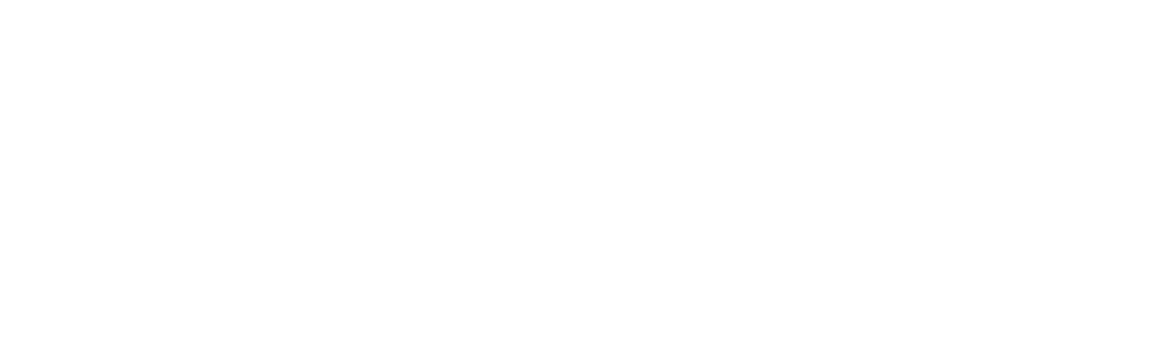Marine systems simulation
FhSim Extras

# SimObjects in fhsim_extras

This library contains SimObjects for visualization and data sharing between programs, primarily for instances of FhSim. Documentation for available SimObjects can be found here.

# Plotting

The SimObject Plot/Scope uses Qt and qcustomplot. The implementation is minimal, but functional: you can plot simulation time series alongside the 3D visualization. Plot/Scope now support the ability to create multiple SimObject plotting instances. Plot/Scope also have the capability to create animation png frames.

## Animations

Suppose you have run a simulation with Plot/Scope, which created a set of animation frames ScopusDdsXXXX.png in folder animations. You can use imagemagick >= 7.0.10-31 to compose an animated png using the following commands, see StackOverflow reference for details. Download and install imagemagick.

cd animations
magick -delay 3.33 -loop 0 'ScopusDds*.png' APNG:AniScopusDds.png

Delay is given in centiseconds, so with 30 frames per second (FPS), delay is

$d = \frac{100}{\text{FPS}} = 3.33.$Animated scopus

## Example

<Contents>
<OBJECTS>
<Lib LibName = "fhsim_extras"
SimObject = "Plot/Scope"
Name = "Scopus"
Width = "300"
Height = "300"
FPS = "30"
DataSamplingFrequency = "30"
TimeWindowSizeSec = "30"
SubplotCount = "2"
Plot1Graphs = "2"
Plot1Legend = "true"
Plot1YLabel = "Length [m]"
P1G1Name = "Estimated"
P1G1LineWidth = "2"
P1G2Name = "Measured"
P1G2LineWidth = "1"
Plot2Graphs = "1"
Plog2Legend = "false"
Plot2YLabel = "Error [m]"
Plot2YRange = "-1,1"
AnimatePng = "true"
AnimateDirectory = "../animations"
AnimateWidth = "300"
AnimateHeight = "300"
AnimateFrequency = "20"
/>
</OBJECTS>
<INTERCONNECTIONS>
<Connection
Scopus.InP1G1="1"
Scopus.InP1G2="2"
Scopus.InP2G1="3"
/>
</INTERCONNECTIONS>
<INITIALIZATION>
<InitialConditions/>
</INITIALIZATION>
<INTEGRATION>
<Engine
IntegratorMethod="2"
NumCores="1"
TOutput="0, 7"
LogStates ="0"
stepsize ="0.01"
HMax="0.2"
HMin="0.00001"
AbsTol="1e-3" RelTol="1e-3"
FileOutput="objects:ports"
/>
</INTEGRATION>
</Contents>

# Data Sharing

The Connection SimObjects are made for data sharing using a simple wrapper around Data Distribution Service OMG DDS. The reader and writer classes are made for sharing double vectors.

• Connection/DdsWriter

It uses ratatosk/interfaces/DdsSignalDllLib.h, which is part ofratatosk-interfaces. The library can be used in other C++ applications too, or directly in e.g. python with pyDDS. This package has a sample application dds-producer that shows how external applications can be used to send data to an FhSim simulation. See the README_dds_producer.md in the playpen binary folder. Please consult SINTEF Ocean and Marine Modeling and Analysis (Karl-Johan Reite) for details on the published data type.

## Example

<Contents>
<OBJECTS>
<Lib LibName = "fhsim_extras"
SimObject = "Connection/DdsWriter"
Name = "DdsOutput"
Partition = "FhSimExternal"
Topic = "PhysicalEntity"
DdsRoot = "../lib"
DdsConfigFile = "../resources/ospl.xml"
ForceDdsEnv = "0"
PortWidth = "2"
ArrayIDs = "1, 2"
PortSizes = "1, 2"
SamplingPeriods = "0.3"
/>
<Lib LibName = "fhsim_extras"
Name = "DdsInput"
Partition = "FhSimExternal"
Topic = "PhysicalEntity"
DdsRoot = "../lib"
DdsConfigFile = "../resources/ospl.xml"
ForceDdsEnv = "1"
InitializationTimeout = "0"
PortWidth = "2"
Tags = "InputAlpha, Input2"
DefaultInputAlpha = "0"
DefaultInput2 = "1, 2"
ArrayIDs = "1, 2"
PortSizes = "1, 2"
/>
</OBJECTS>
<INTERCONNECTIONS>
<Connection
DdsOutput.In1="1"
DdsOutput.In2="2, 3"
/>
</INTERCONNECTIONS>
<INITIALIZATION>
<InitialConditions/>
</INITIALIZATION>
<INTEGRATION>
<Engine
IntegratorMethod="2"
NumCores="1"
TOutput="0, 5, 5"
LogStates ="1"
stepsize ="0.001"
HMax="0.2"
HMin="0.00001"
AbsTol="1e-3" RelTol="1e-3"
FileOutput="object.DdsInput:ports"
/>
</INTEGRATION>
</Contents>

# SimObjects in fhsim_extras

This library contains SimObjects for visualization and data sharing between programs, primarily for instances of FhSim. Documentation for available SimObjects can be found under modules.

# Plotting

The SimObject Plot/Scope uses Qt and qcustomplot. The implementation is minimal, but functional: you can plot simulation time series alongside the 3D visualization. Plot/Scope now support the ability to create multiple SimObject plotting instances. Plot/Scope also have the capability to create animation png frames.

## Animations

Suppose you have run a simulation with Plot/Scope, which created a set of animation frames ScopusDdsXXXX.png in folder animations. You can use imagemagick >= 7.0.10-31 to compose an animated png using the following commands, see StackOverflow reference for details. Download and install imagemagick.

cd animations
magick -delay 3.33 -loop 0 'ScopusDds*.png' APNG:AniScopusDds.png

Delay is given in centiseconds, so with 30 frames per second (FPS), delay is

$d = \frac{100}{\text{FPS}} = 3.33.$Animated scopus

## Example

<Contents>
<OBJECTS>
<Lib LibName = "fhsim_extras"
SimObject = "Plot/Scope"
Name = "Scopus"
Width = "300"
Height = "300"
FPS = "30"
DataSamplingFrequency = "30"
TimeWindowSizeSec = "30"
SubplotCount = "2"
Plot1Graphs = "2"
Plot1Legend = "true"
Plot1YLabel = "Length [m]"
P1G1Name = "Estimated"
P1G1LineWidth = "2"
P1G2Name = "Measured"
P1G2LineWidth = "1"
Plot2Graphs = "1"
Plog2Legend = "false"
Plot2YLabel = "Error [m]"
Plot2YRange = "-1,1"
AnimatePng = "true"
AnimateDirectory = "../animations"
AnimateWidth = "300"
AnimateHeight = "300"
AnimateFrequency = "20"
/>
</OBJECTS>
<INTERCONNECTIONS>
<Connection
Scopus.InP1G1="1"
Scopus.InP1G2="2"
Scopus.InP2G1="3"
/>
</INTERCONNECTIONS>
<INITIALIZATION>
<InitialConditions/>
</INITIALIZATION>
<INTEGRATION>
<Engine
IntegratorMethod="2"
NumCores="1"
TOutput="0, 7"
LogStates ="0"
stepsize ="0.01"
HMax="0.2"
HMin="0.00001"
AbsTol="1e-3" RelTol="1e-3"
FileOutput="objects:ports"
/>
</INTEGRATION>
</Contents>

# Data Sharing

The Connection SimObjects are made for data sharing using a simple wrapper around Data Distribution Service OMG DDS. The reader and writer classes are made for sharing double vectors.

It uses ratatosk/interfaces/DdsSignalDllLib.h, which is part ofratatosk-interfaces. The library can be used in other C++ applications too, or directly in e.g. python with pyDDS. This package has a sample application dds-producer that shows how external applications can be used to send data to an FhSim simulation. See the README_dds_producer.md in the playpen binary folder. Please consult SINTEF Ocean and Marine Modeling and Analysis (Karl-Johan Reite) for details on the published data type.

## Example

<Contents>
<OBJECTS>
<Lib LibName = "fhsim_extras"
SimObject = "Connection/DdsWriter"
Name = "DdsOutput"
Partition = "FhSimExternal"
Topic = "PhysicalEntity"
DdsRoot = "../lib"
DdsConfigFile = "../resources/ospl.xml"
ForceDdsEnv = "0"
PortWidth = "2"
ArrayIDs = "1, 2"
PortSizes = "1, 2"
SamplingPeriods = "0.3"
/>
<Lib LibName = "fhsim_extras"
Name = "DdsInput"
Partition = "FhSimExternal"
Topic = "PhysicalEntity"
DdsRoot = "../lib"
DdsConfigFile = "../resources/ospl.xml"
ForceDdsEnv = "1"
InitializationTimeout = "0"
PortWidth = "2"
Tags = "InputAlpha, Input2"
DefaultInputAlpha = "0"
DefaultInput2 = "1, 2"
ArrayIDs = "1, 2"
PortSizes = "1, 2"
/>
</OBJECTS>
<INTERCONNECTIONS>
<Connection
DdsOutput.In1="1"
DdsOutput.In2="2, 3"
/>
</INTERCONNECTIONS>
<INITIALIZATION>
<InitialConditions/>
</INITIALIZATION>
<INTEGRATION>
<Engine
IntegratorMethod="2"
NumCores="1"
TOutput="0, 5, 5"
LogStates ="1"
stepsize ="0.001"
HMax="0.2"
HMin="0.00001"
AbsTol="1e-3" RelTol="1e-3"